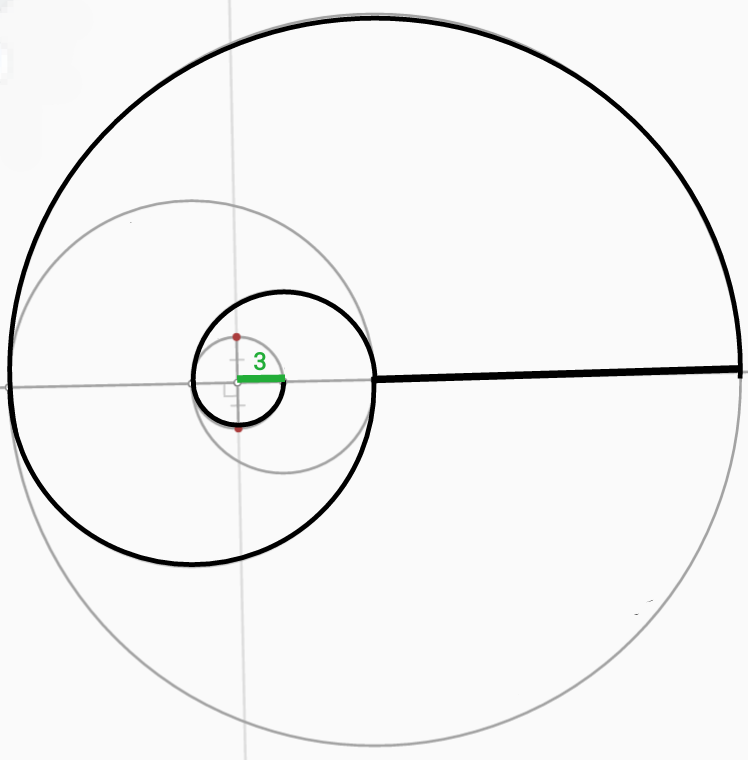# Cutie Shells! (Arc Calculation I)

Geometry Level 1

Add up the black bold lines' lengths and type the total as the answer.

Assume that all angles that look perpendicular to be perpendicular, and all arcs that look like part of a circle to be part of a circle. All assumable intersections are in fact exact intersections, and should be taken as such.

$\text{Fractions should be converted as decimals.}$

$\text{If it is a recurring decimal, type all the decimals until the recursion stops.}$

Assume that $π$ is equal to $\frac{22}{7}$$\color{#333333}\text{Whoever wants to submit a report for this problem can go here}$

×